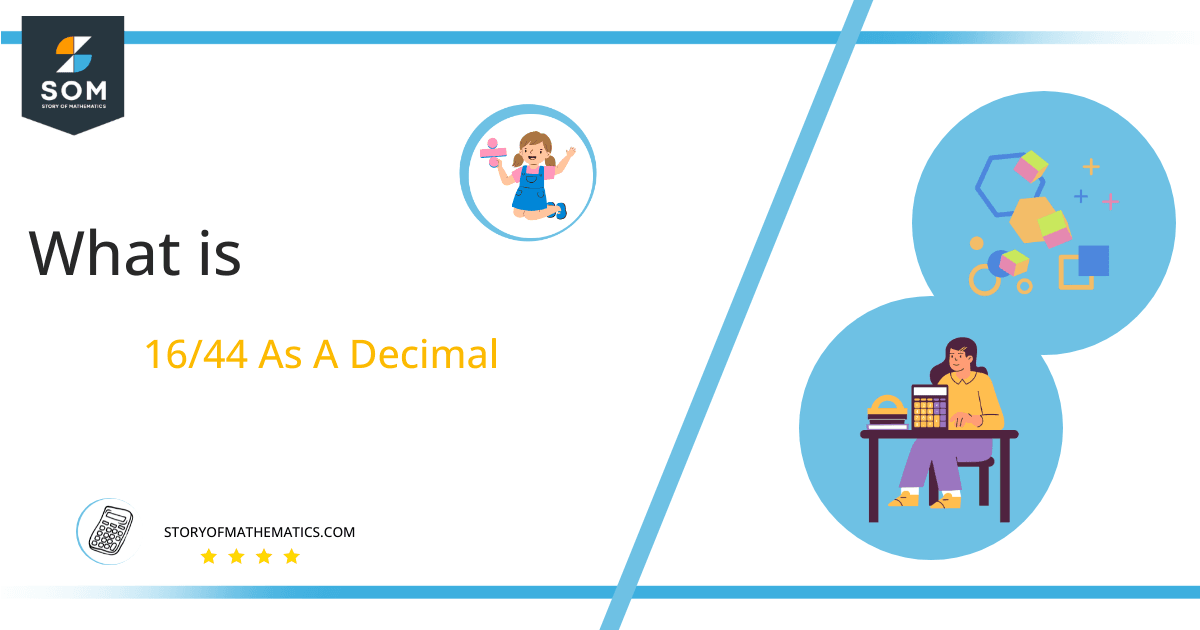# What Is 16/44 as a Decimal + Solution With Free Steps

The fraction 16/44 as a decimal is equal to 0.363.

The a/b form can represent fractions, with a and b as the numerator and denominator, respectively. The division is one of the most challenging mathematical operations because it is required when working with fractions. But if we employ the approach that will be covered later, we can simplify it.

Here, we are more interested in the division types that result in a Decimal value, as this can be expressed as a Fraction. We see fractions as a way of showing two numbers having the operation of Division between them that result in a value that lies between two Integers.Now, we introduce the method used to solve said fraction to decimal conversion, called Long Division, which we will discuss in detail moving forward. So, let’s go through the Solution of fraction 16/44. The following diagram shows the long division procedure:Figure 1

## Solution

First, we convert the fraction components, i.e., the numerator and the denominator, and transform them into the division constituents, i.e., the Dividend and the Divisor, respectively.

This can be done as follows:

Dividend = 16

Divisor = 44

Now, we introduce the most important quantity in our division process: the Quotient. The value represents the Solution to our division and can be expressed as having the following relationship with the Division constituents:

Quotient = Dividend $\div$ Divisor = 16 $\div$ 44

This is when we go through the Long Division solution to our problem.

## 16/44 Long Division Method

We start solving a problem using the Long Division Method by first taking apart the division’s components and comparing them. As we have 16 and 44, we can see how 16 is Smaller than 44, and to solve this division, we require that 16 be Bigger than 44.

This is done by multiplying the dividend by 10 and checking whether it is bigger than the divisor or not. If so, we calculate the Multiple of the divisor closest to the dividend and subtract it from the Dividend. This produces the Remainder, which we then use as the dividend later.

Now, we begin solving for our dividend 16, which after getting multiplied by 10 becomes 160.

We take this 160 and divide it by 44; this can be done as follows:

160 $\div$ 44 $\approx$ 3

Where:

44 x 3 = 132

This will lead to the generation of a Remainder equal to 160 – 132 = 28. Now this means we have to repeat the process by Converting the 28 into 280 and solving for that:

280 $\div$ 44 $\approx$ 6

Where:

44 x 6 = 264

This, therefore, produces another Remainder which is equal to 280 – 262 = 16. Now we must solve this problem to Third Decimal Place for accuracy, so we repeat the process with dividend 160.

160 $\div$ 44 $\approx$ 3

Where:

44 x 3 = 132

Finally, we have a Quotient generated after combining the three pieces of it as 0.363=z, with a Remainder equal to 28.Images/mathematical drawings are created with GeoGebra.